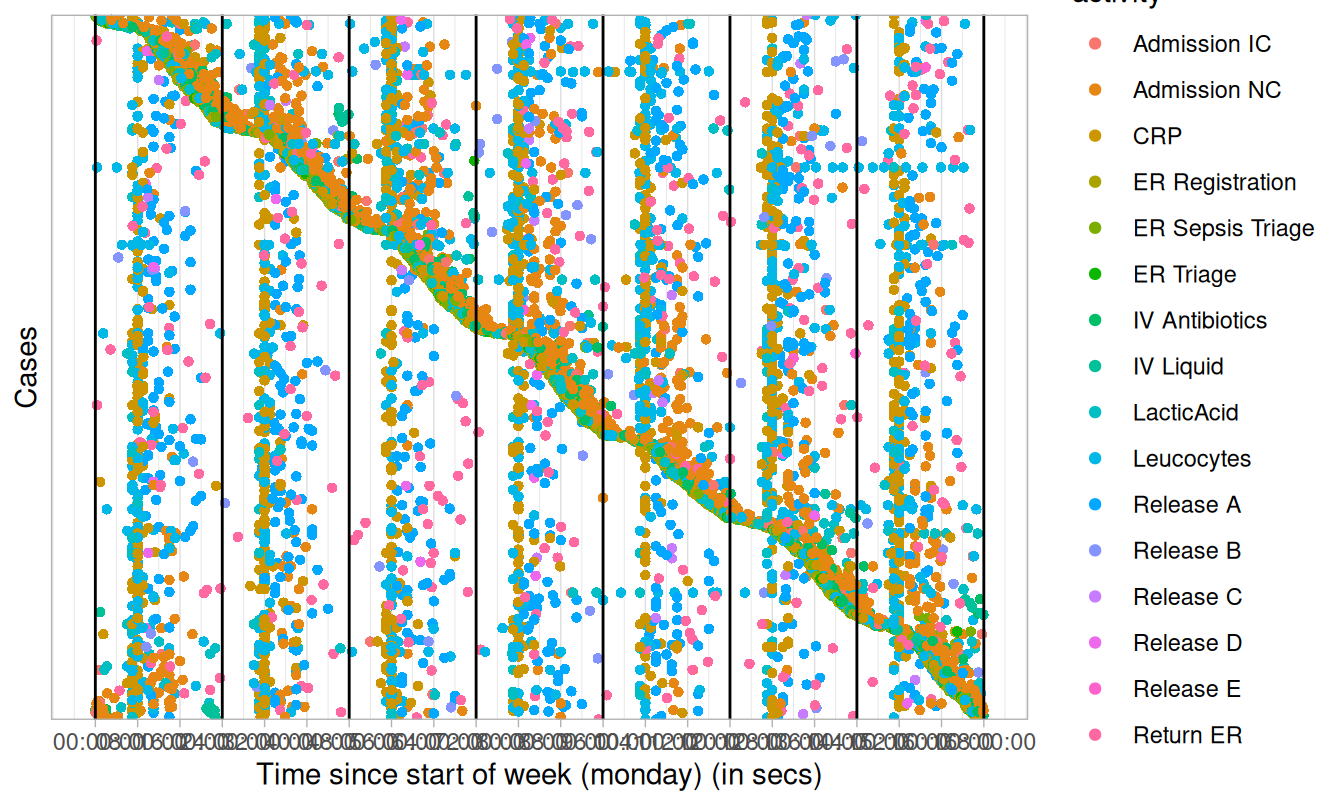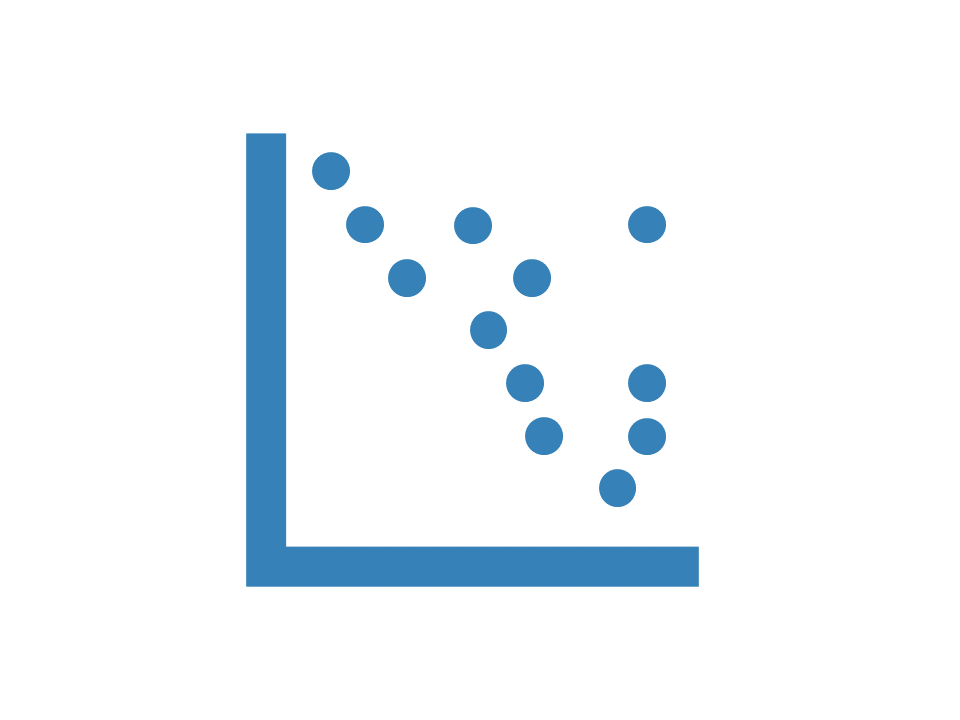# Dotted chart

library(bupaverse)

Dotted charts can be made with dotted_chart(). A dotted chart is a graph in which each activity instance is displayed by a dot. The x-axis refers to the time aspect, while the y-axis refers to cases. The dotted chart function has 3 arguments:

• x:
• absolute: absolute time on x-axis
• relative: time difference since start case on x-axis
• relative_day: time difference since start of day on x-axis (00:00)
• relative_week: time difference since start of week on x-axis (Mondag 00:00)
• sort: The ordering of the cases along the y-axis: start, end, duration, start_day, or start_week. Value auto (default) will set sort appropriately given the argument for x
• color: The attribute used to color the activity instances. Defaults to the activity type.

Below, you can see some examples for dotted charts with various configurations.

## Absolute

sepsis %>%
dotted_chart(x = "absolute")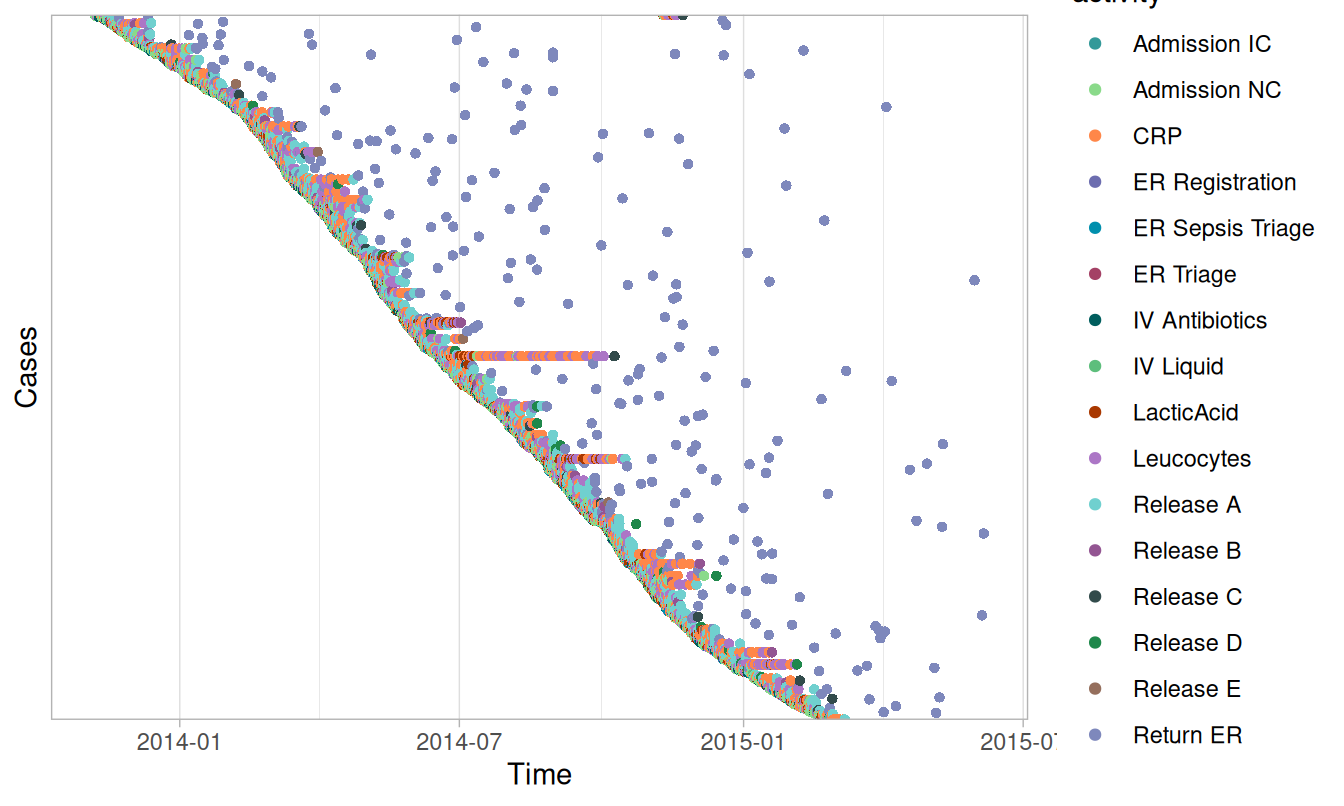sepsis %>%
dotted_chart(x = "absolute", sort = "end")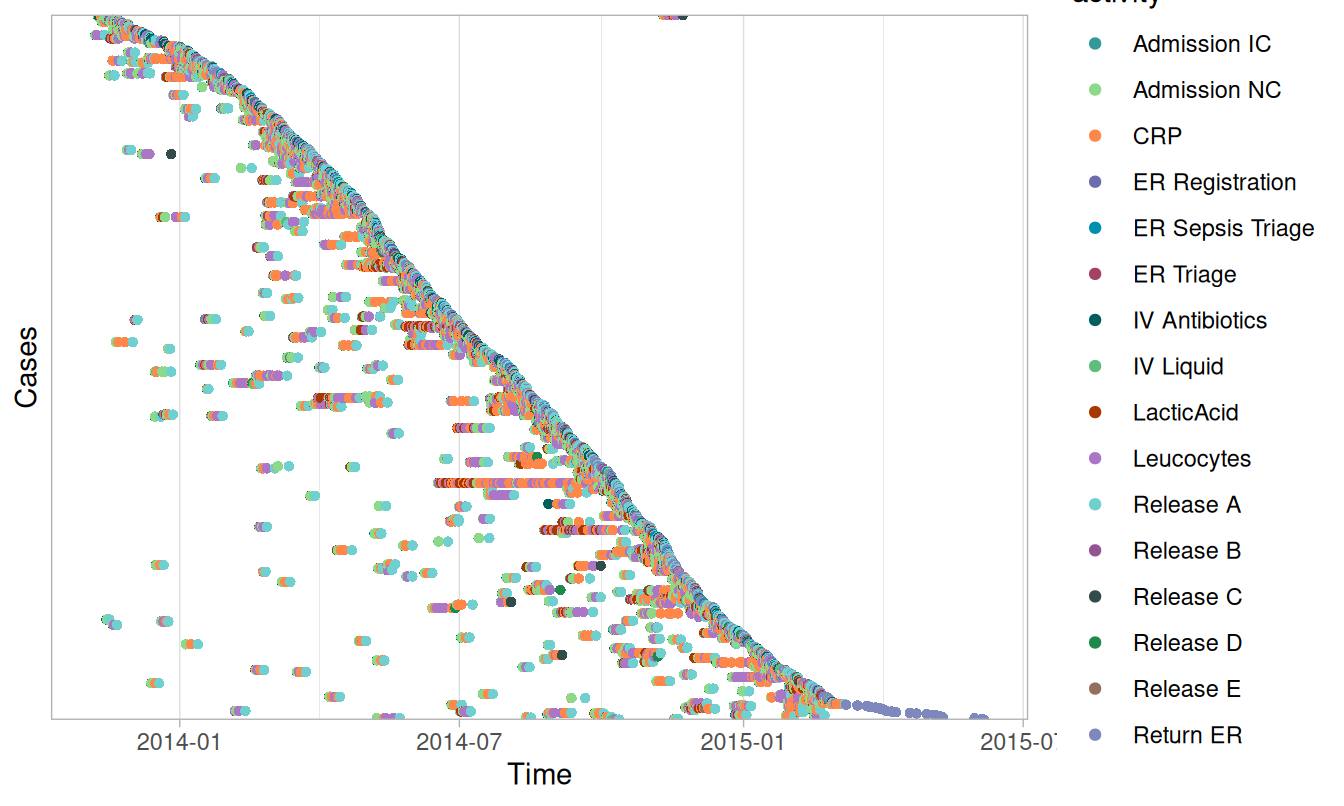## Relative

sepsis %>%
dotted_chart(x = "relative")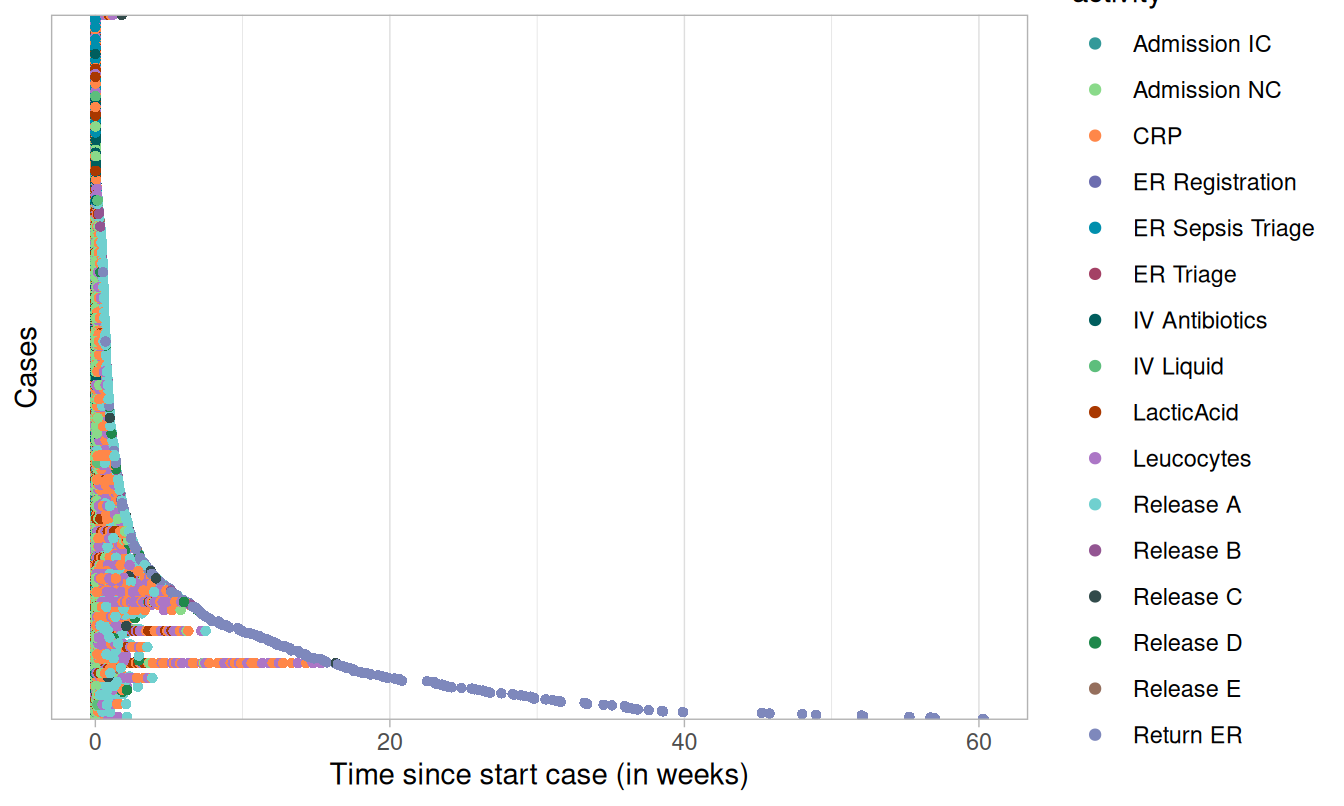## Relative day

sepsis %>%
dotted_chart(x = "relative_day")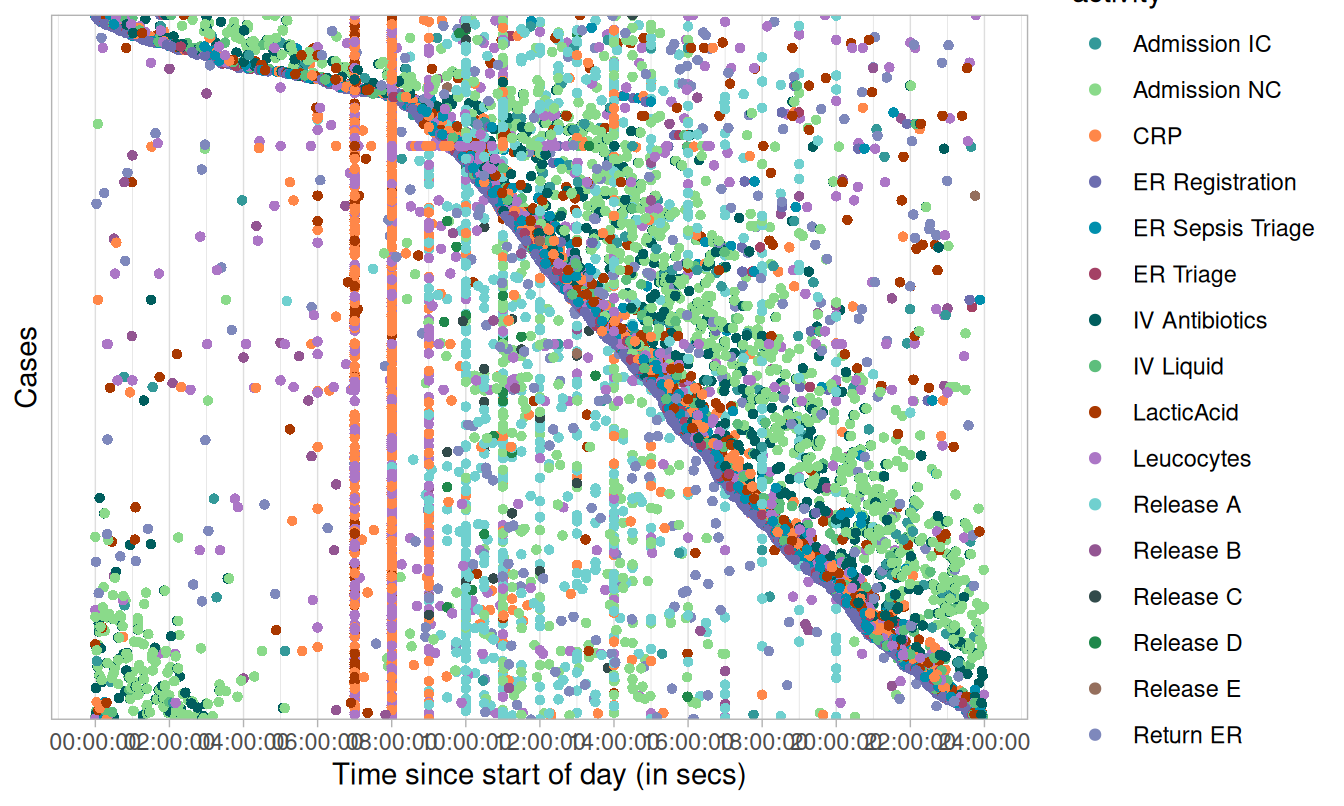## Relative week

sepsis %>%
dotted_chart(x = "relative_week")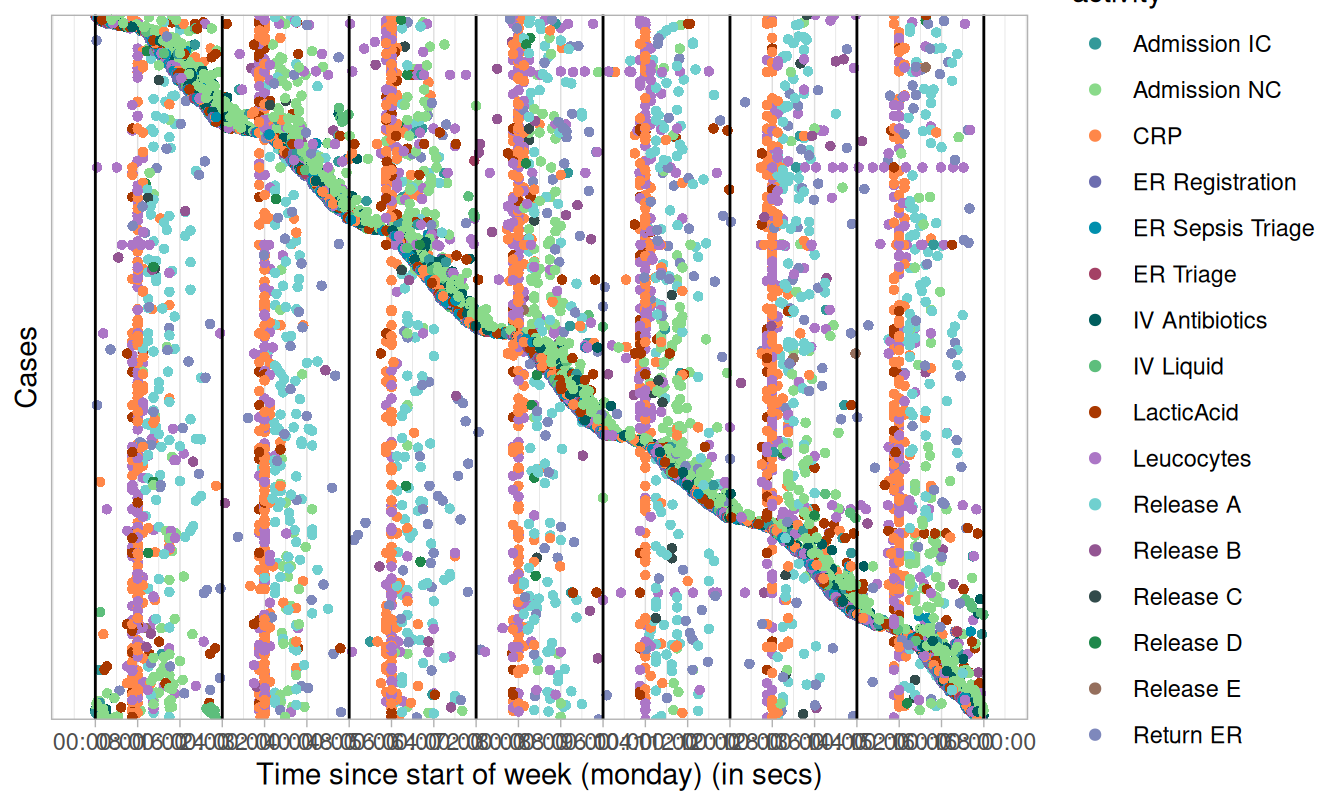Other configurations:

• change the used color scale by configuring the scale_color argument.
sepsis %>%
dotted_chart(x = "relative_week",
scale_color = ggplot2::scale_color_discrete)